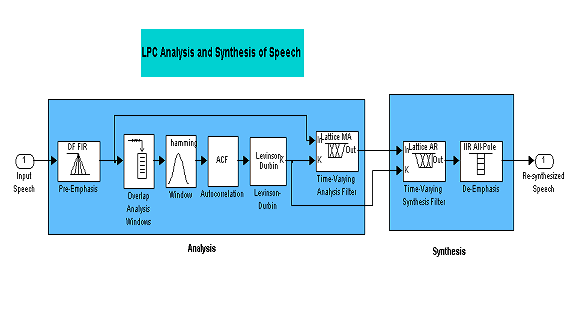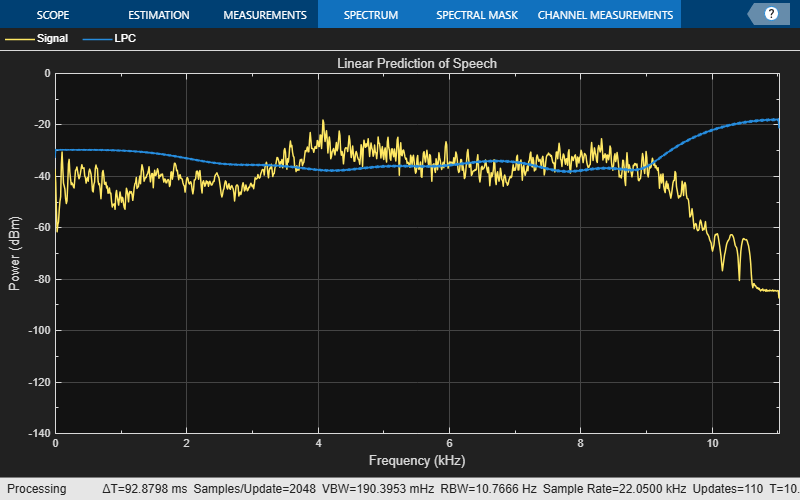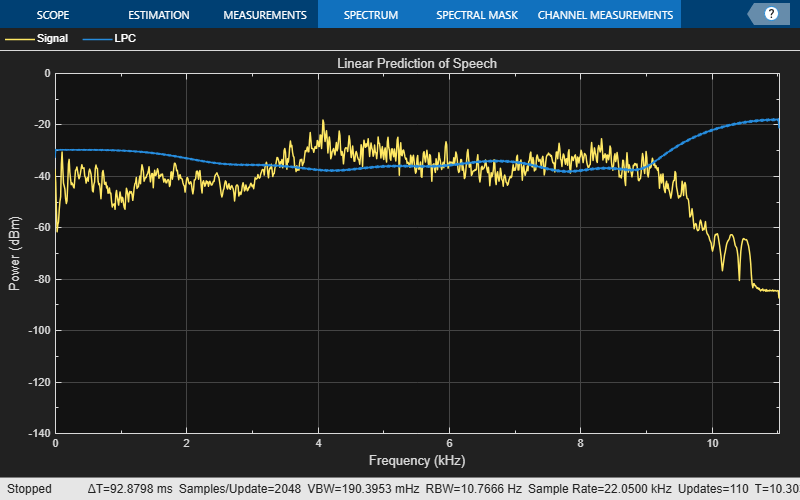# LPC Analysis and Synthesis of Speech

This example shows how to implement a speech compression technique known as Linear Prediction Coding (LPC) using DSP System Toolbox™ functionality available at the MATLAB® command line.

### Introduction

In this example you implement LPC analysis and synthesis (LPC coding) of a speech signal. This process consists of two steps: analysis and synthesis. In the analysis section, you extract the reflection coefficients from the signal and use it to compute the residual signal. In the synthesis section, you reconstruct the signal using the residual signal and reflection coefficients. The residual signal and reflection coefficients require less number of bits to code than the original speech signal.

The block diagram below shows the system you will implement.In this simulation, the speech signal is divided into frames of size 3200 samples, with an overlap of 1600 samples. Each frame is windowed using a Hamming window. Twelfth-order autocorrelation coefficients are found, and then the reflection coefficients are calculated from the autocorrelation coefficients using the Levinson-Durbin algorithm. The original speech signal is passed through an analysis filter, which is an all-zero filter with coefficients as the reflection coefficients obtained above. The output of the filter is the residual signal. This residual signal is passed through a synthesis filter which is the inverse of the analysis filter. The output of the synthesis filter is the original signal.

### Initialization

Here you initialize some of the variables like the frame size and also instantiate the System objects used in your processing. These objects also pre-compute any necessary variables or tables resulting in efficient processing calls later inside a loop.

Initialize variables.

```frameSize = 1600; fftLen = 2048;```

Here you create a System object™ to read from an audio file and determine the file's audio sampling rate.

```audioReader = dsp.AudioFileReader('SamplesPerFrame', frameSize, ... 'OutputDataType', 'double'); fileInfo = info(audioReader); Fs = fileInfo.SampleRate;```

Create an FIR digital filter System object used for pre-emphasis.

```preEmphasisFilter = dsp.FIRFilter(... 'Numerator', [1 -0.95]);```

Create a buffer System object and set its properties such that you get an output of twice the length of the `frameSize` with an overlap length of `frameSize`.

`signalBuffer = dsp.AsyncBuffer(2*frameSize);`

Create an FIR digital filter System object used for analysis. Also create two all-pole digital filter System objects used for synthesis and de-emphasis.

```analysisFilter = dsp.FIRFilter(... 'Structure','Lattice MA',... 'ReflectionCoefficientsSource', 'Input port'); synthesisFilter = dsp.AllpoleFilter('Structure','Lattice AR'); deEmphasisFilter = dsp.AllpoleFilter('Denominator',[1 -0.95]);```

Create a System object to play the resulting audio.

```audioWriter = audioDeviceWriter('SampleRate', Fs); % Setup plots for visualization. scope = spectrumAnalyzer('SampleRate', Fs, ... 'PlotAsTwoSidedSpectrum', false, 'YLimits', [-140, 0], ... 'Title', 'Linear Prediction of Speech', ... 'ShowLegend', true, 'ChannelNames', {'Signal', 'LPC'});```

### Stream Processing Loop

Here you call your processing loop where you do the LPC analysis and synthesis of the input audio signal using the System objects you have instantiated.

The loop is stopped when you reach the end of the input file, which is detected by the `AudioFileReader` System object.

```while ~isDone(audioReader) % Read audio input sig = audioReader(); % Analysis % Note that the filter coefficients are passed in as an argument to the % analysisFilter System object. sigpreem = preEmphasisFilter(sig); write(signalBuffer,sigpreem); sigbuf = read(signalBuffer,2*frameSize, frameSize); hammingwin = hamming(2*frameSize); sigwin = hammingwin.*sigbuf; % Autocorrelation sequence on [0:13] sigacf = xcorr(sigwin, 12, 'biased'); sigacf = sigacf(13:end); % Compute the reflection coefficients from auto-correlation function % using the Levinson-Durbin recursion. The function outputs both % polynomial coefficients and reflection coefficients. The polynomial % coefficients are used to compute and plot the LPC spectrum. [sigA, ~, sigK] = levinson(sigacf); % Levinson-Durbin siglpc = analysisFilter(sigpreem, sigK); % Synthesis synthesisFilter.ReflectionCoefficients = sigK.'; sigsyn = synthesisFilter(siglpc); sigout = deEmphasisFilter(sigsyn); % Play output audio audioWriter(sigout); % Update plots sigA_padded = zeros(size(sigwin), 'like', sigA.'); % Zero-padded to plot sigA_padded(1:size(sigA.',1), :) = sigA.'; scope([sigwin, sigA_padded]); end```### Release

Here you call the release method on the System objects to close any open files and devices.

```release(audioReader); pause(10*audioReader.SamplesPerFrame/audioReader.SampleRate); % Wait until audio finishes playing release(audioWriter); release(scope);```### Conclusion

You have seen here the implementation of speech compression technique using Linear Prediction Coding. The implementation used the DSP System Toolbox functionality available at the MATLAB command line. The code involves only calling of the successive System objects with appropriate input arguments. This involves no error prone manual state tracking which may be the case for instance for a MATLAB implementation of Buffer.